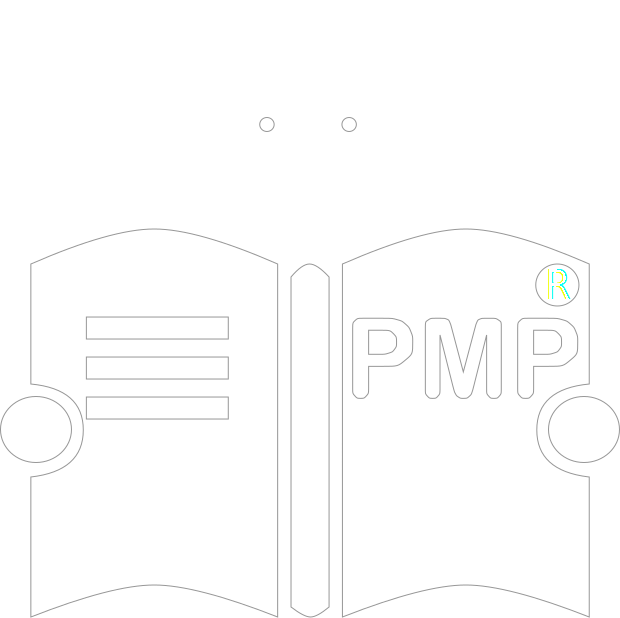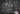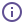# PMP formulas you have to knowWhen taking the PMP exam, you can expect to answer different types of questions: formula-based questions, situation-based questions, definition-based questions, ITTO-based questions, interpretational questions and PMP ethics questions.

Different types of questions need a different approach. For me personally, it's easier to calculate, and formula-based questions were my favorite during the preparation for the exam. I made a list of all formulas used in project management and learned them by heart. I will share this list and also, I will show you my cheat sheet of the formulas that I actually used during the exam.

PV = Planned % Complete * BACWhat the project should be worthPV - planned value
BAC - budget at completion
EV = Actual % Complete * BACWhat the project is worthEV - earned value
BAC - budget at completion
SD = (P - O) / 6SD measures the Variation from AverageSD - standard deviation
P - pessimistic estimate
O - optimistic estimate
EAD = (O + 4R + P) / 6EAD calculated by beta distributionEAD - estimated activity duration
R - realistic estimate
P - pessimistic estimate
O - optimistic estimate
EAD = (O + R + P) / 3EAD calculated by triangular distributionEAD - estimated activity duration
R - realistic estimate
P - pessimistic estimate
O - optimistic estimate
CV = EV - ACCV represents the amount of budget deficit or surplus at a given point in timeCV - cost variance
EV - earned value
AC - actual costSV = EV - PVSV aims to measure schedule performance through the difference between the earned value and the planned valueSV - schedule variance
EV - earned value
PV - planned valueCPI = EV / ACCPI measures the cost efficiency of budgeted resourcesCPI - cost performance index
EV - earned value
AC - actual costSPI = EV / PVSPI measures schedule efficiencySPI - schedule performance index
EV - earned value
PV - planned valueETC = EAC - ACETC predicts how much more the remainder of the project will costETC - estimate to complete
EAC - estimate at completion
AC - actual cost
EAC = BAC / CPIPredicts final project costs based on current performanceEAC - estimate at completion
BAC - budget at completion
CPI - cost performance index
EAC = AC + BAC - EVPredicts final project costs based on current performanceEAC - estimate at completion
BAC - budget at completion
AC - actual cost
EV - earned value
EAC = AC + [(BAC - EV) / (CPI * SPI)]Predicts final project costs based on current performanceEAC - estimate at completion
BAC - budget at completion
AC - actual cost
EV - earned value
CPI - cost performance index
SPI - schedule performance index
VAC = BAC - EACVAC is a projection of the amount of budget deficit or surplusVAC - variance at completion
BAC - budget at completion
EAC - estimate at completionTCPI = (BAC - EV) / (BAC - AC)Predicts likelihood of reaching BACTCPI - to-complete performance index
BAC - budget at completion
EV - earned value
AC - actual costTCPI = (BAC - EV) / (EAC - AC)Predicts likelihood of reaching EACTCPI - to-complete performance index
BAC - budget at completion
EV - earned value
AC - actual cost
EAC - estimate at completionN * (N - 1) / 2Number of communication channelsN - number of team membersSlack = LS - ESDate constraints, within which we can plan our activity without affecting the overall length of the projectLS - late start
ES - early start
Slack = LF - EFDate constraints, within which we can plan our activity without affecting the overall length of the projectLF - late finish
EF - early finish
EF = ES + Duration - 1The earliest you can finish the activity without delaying the project end dateEF - early finish
ES - early start
LS = LF - Duration + 1The latest you can start the activity without delaying the project end dateLS - late start
LF - late finish
EMV = Probability * ImpactEMV represents the expected money to be made from a specific decisionEMV - expected monetary value
ROI = (Net Profit / COI) * 100Measures profit or loss gained through an investment based on the amount investedROI - return on investment
COI - cost of investment
CBR = NPV / initial investment costCBR tells about the profit to be received from an investmentCBR - cost-benefit ratio
NPV - net present value
NPV = Value / (1 + r) ^ tNet present value of investmentValue - value of benefits
r - rate of discount
t - defined time frame

This is the most complete list of formulas I could make for myself. I knew all of them and I definitely recommend you learning them all without exception. However, some of them are used more often than others.

On the PMP exam, it's highly effective to write a cheat sheet as soon as the exam starts. It's a concise set of notes used for quick reference. In my cheat sheet I included the most commonly used formulas. From my personal experience, all the formulas that I needed on the exam were on this list. But this is only my experience and you never know what sorts of questions you will have and what formulas you will have to use. That's why, even though most probably you will use the formulas mentioned in my cheat sheet, you should not limit yourself to only them, but learn all the formulas used in project management.

## PMP Formulas Cheat Sheet

• CV = EV - AC
• SV = EV - PV
• EAC = BAC / CPI
• VAC = BAC - EAC
• CPI = EV / AC
• SPI = EV / PV
• ETC = EAC - AC
• TCPI = (BAC - EV) / (BAC - AC)
• PV = Planned % Complete * BAC
• EV = Actual % Complete * BAC
Author: Yury Shkoda
Date: 4/8/2020